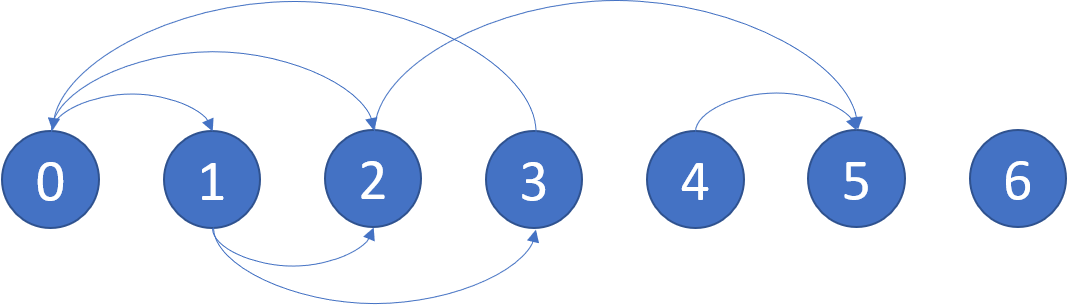## Algorithm

Problem Name: 802. Find Eventual Safe States

There is a directed graph of `n` nodes with each node labeled from `0` to `n - 1`. The graph is represented by a 0-indexed 2D integer array `graph` where `graph[i]` is an integer array of nodes adjacent to node `i`, meaning there is an edge from node `i` to each node in `graph[i]`.

A node is a terminal node if there are no outgoing edges. A node is a safe node if every possible path starting from that node leads to a terminal node (or another safe node).

Return an array containing all the safe nodes of the graph. The answer should be sorted in ascending order.

Example 1:```Input: graph = [[1,2],[2,3],,,,[],[]]
Output: [2,4,5,6]
Explanation: The given graph is shown above.
Nodes 5 and 6 are terminal nodes as there are no outgoing edges from either of them.
Every path starting at nodes 2, 4, 5, and 6 all lead to either node 5 or 6.```

Example 2:

```Input: graph = [[1,2,3,4],[1,2],[3,4],[0,4],[]]
Output: 
Explanation:
Only node 4 is a terminal node, and every path starting at node 4 leads to node 4.
```

Constraints:

• `n == graph.length`
• `1 <= n <= 104`
• `0 <= graph[i].length <= n`
• `0 <= graph[i][j] <= n - 1`
• `graph[i]` is sorted in a strictly increasing order.
• The graph may contain self-loops.
• The number of edges in the graph will be in the range `[1, 4 * 104]`.

## Code Examples

### #1 Code Example with Javascript Programming

```Code - Javascript Programming```

``````
class Solution {
public:
vector eventualSafeNodes(vector>& graph) {
vectorres;
int n = graph.size();
vectorloop(n), safe(n), visited(n);
for(int i = 0; i < n; i++)
if(isSafe(graph, visited, loop, safe, i)) res.push_back(i);
return res;
}

bool isSafe(vector>& graph, vector& visited, vector& loop, vector& safe, int node){
if(safe[node]) return true;
if(loop[node] || visited[node]) return false;
visited[node] = 1;
bool b = true;
for(int neigh: graph[node]) b &= isSafe(graph, visited, loop, safe, neigh);
visited[node] = 0;
b ? safe[node] = 1 : loop[node] = 1;
return b;
}
};
``````
Copy The Code &

Input

cmd
graph = [[1,2],[2,3],,,,[],[]]

### #2 Code Example with Python Programming

```Code - Python Programming```

``````
class Solution:
def eventualSafeNodes(self, graph):
def explore(i):
visited[i] = 0
for v in graph[i]:
if visited[v] == 0 or (visited[v] == -1 and explore(v)): return True
visited[i] = 1
res.append(i)
return False
visited, res = [-1] * len(graph), []
for i in range(len(graph)):
if visited[i] == -1: explore(i)
return sorted(res)
``````
Copy The Code &

Input

cmd
graph = [[1,2],[2,3],,,,[],[]]

Output

cmd
[2,4,5,6][2,4,5,6]